﻿ Supersonic flow around an 2-D NACA airfoil - XSim

# Supersonic flow around an 2-D NACA airfoil

Update: November 26, 2018
OpenFOAM 4.x

## Case directory

\$FOAM_TUTORIALS/compressible/sonicFoam/ras/nacaAirfoil

## Summary

We calculate the supersonic air flow (Mach number is about 1.78) around a NACA airfoil.

The flow is outflowing at (600, 148.16) m/s from the region "OUTL2" behind the airfoil, and free-flowing in the other region "INLE1", and is calculated for 0.01 second. The calculation is performed as a 2-dimensional analysis.Model geometry

Launder-Sharma low Reynolds number k-ε model is used as the turbulence model.

We also calculates the coefficient of torque, coefficient of drag, coefficient of lift, etc. by setting the file system/controlDict as follows. Each value is output to the file postProcessing/forces/0/forceCoeffs.dat.

```functions
{
forces
{
type            forceCoeffs;
libs ( "libforces.so" );
writeControl   timeStep;
writeInterval  1;

patches
(
WALL10
);

log         true;
rhoInf      1;
CofR        (0 0 0);
liftDir     (-0.239733 0.970839 0);
dragDir     (0.970839 0.239733 0);
pitchAxis   (0 0 1);
magUInf     618.022;
lRef        1;
Aref        1;
}
}
```

We use the mesh that are converted from the Star-CD (v3) pro-STAR format mesh.

The meshes are as follows, and the number of mesh is 40000.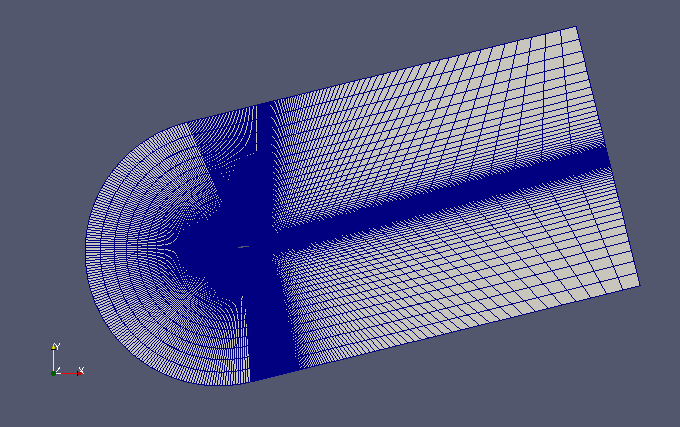Entire mesh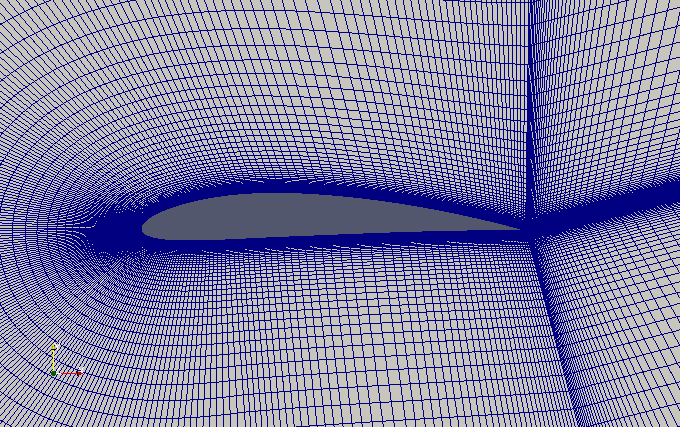Mesh around the airfoil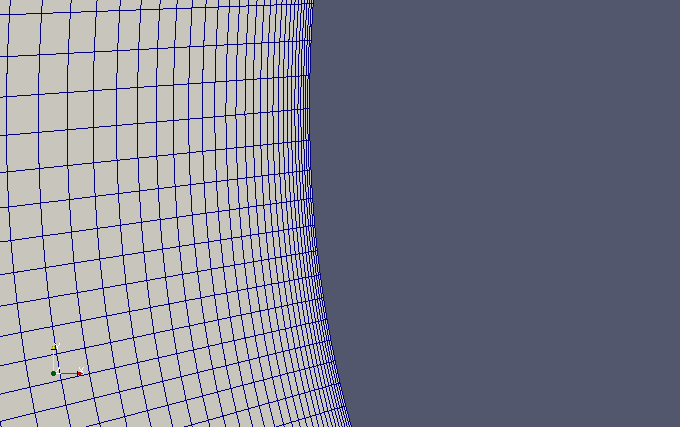Zoomed mesh at the leading edge of the airfoil

The calculation result is as follows.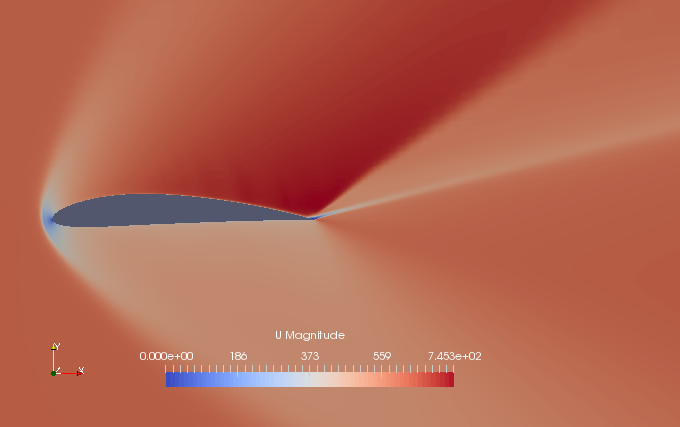Flow velocity at final time (U)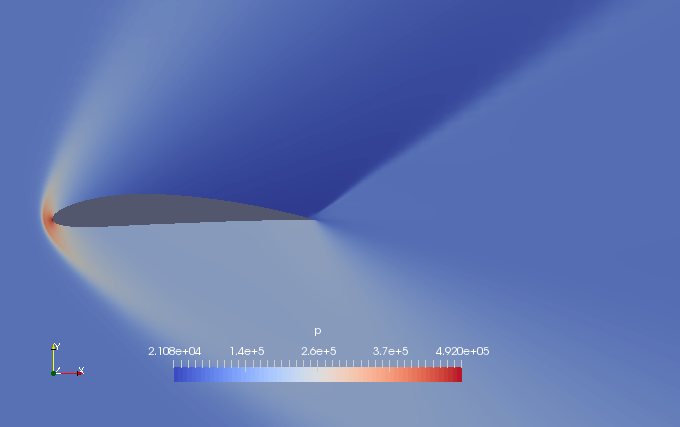Pressure at final time (p)

The file postProcessing/forces/0/forceCoeffs.dat will have the data as shown below.

```# Force coefficients
# liftDir    : (-2.397330e-01 9.708390e-01 0.000000e+00)
# dragDir    : (9.708390e-01 2.397330e-01 0.000000e+00)
# pitchAxis  : (0.000000e+00 0.000000e+00 1.000000e+00)
# magUInf    : 6.180220e+02
# lRef       : 1.000000e+00
# Aref       : 1.000000e+00
# CofR       : (0.000000e+00 0.000000e+00 0.000000e+00)
# Time       	Cm           	Cd           	Cl           	Cl(f)        	Cl(r)
4e-08        	1.646333e-301	1.332445e-301	2.609097e-301	2.950882e-301	-3.417852e-302
8e-08        	2.941511e-301	2.299226e-301	4.730491e-301	5.306757e-301	-5.762652e-302

…………

0.00999992   	3.930796e-301	2.814022e-301	6.597210e-301	7.229401e-301	-6.321912e-302
0.00999996   	3.930795e-301	2.814021e-301	6.597209e-301	7.229400e-301	-6.321906e-302
0.01         	3.930795e-301	2.814020e-301	6.597209e-301	7.229400e-301	-6.321902e-302
```

where Cm is the coefficient of torque, Cd is the coefficient of drag, Cf is the coefficient of lift, Cl(f) is Cl/2.0 + Cm, and Cl(r) is Cl/2.0 - Cm.

## Commands

cp -r \$FOAM_TUTORIALS/compressible/sonicFoam/ras/nacaAirfoil nacaAirfoil
cd nacaAirfoil

star3ToFoam prostar/nacaAirfoil
sed -i -e 's/symmetry\([)]*;\)/empty\1/' constant/polyMesh/boundary
sonicFoam

paraFoam

The command "star3ToFoam" converts the mesh from Star-CD (v3) pro-STAR format to OpenFOAM format, and the command "sed" changes the boundary condition of the region "SYMP3" from "symmetric" to "empty".

## Calculation time

5 hours 27 minutes 58 seconds *Single, Inter(R) Core(TM) i7-8700 CPU @ 3.20GHz 3.19GHz